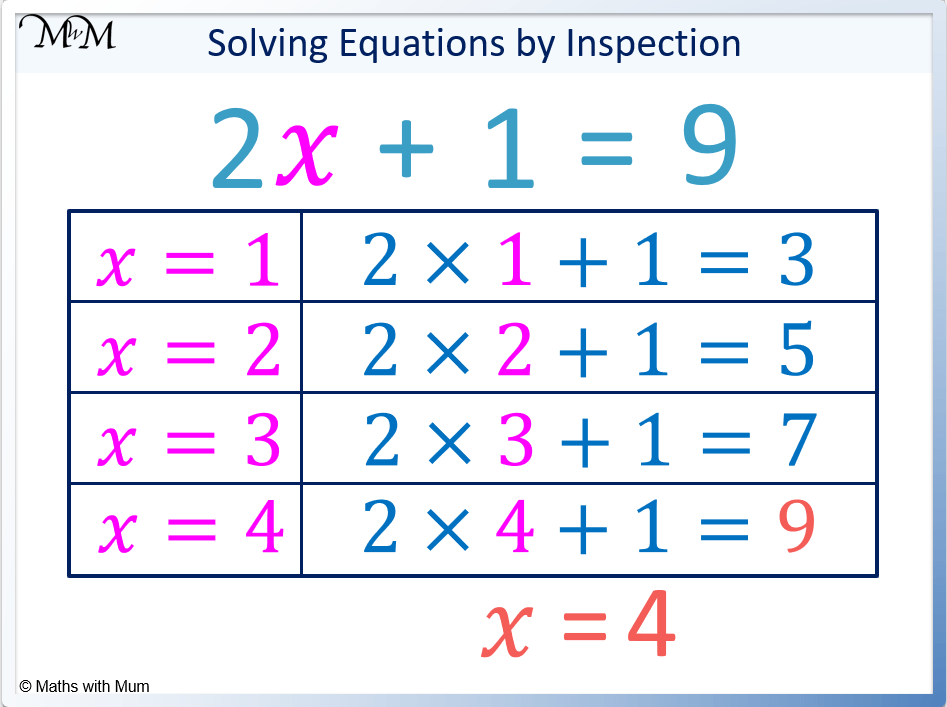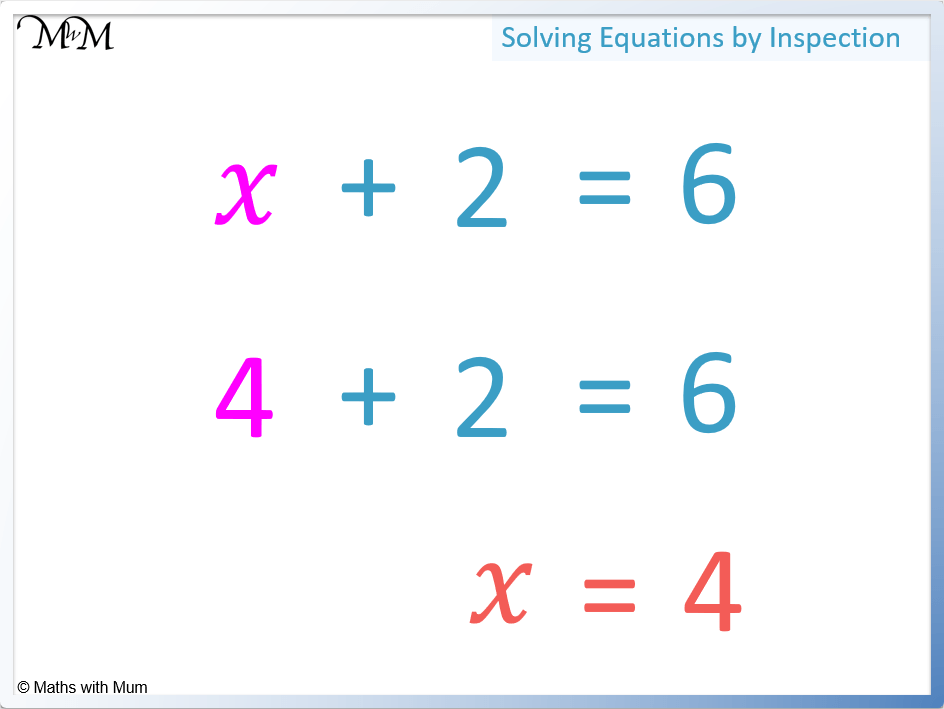# How to Solve Equations by Inspection

How to Solve Equations by InspectionTo solve an equation by inspection, just think what number must be placed there to obtain the correct answer.• Solving an equation means to find out the value of the variable (letter) which makes the equation equal to the value given.
• In the equation 𝑥 + 4 = 8, we think of the number that makes 8 when we add 4 to it. Since 4 + 4 = 8, 𝑥 must be equal to 4.
• In the equation 𝑥 – 3 = 4, we think of the number that makes 4 when we take away 3 from it. Since 7 – 3 = 4, 𝑥 must be equal to 7.
• In the equation 9 – 𝑥 = 8, we think of the number that we subtract from 9 to make 8. Since 9 – 1 = 8, 𝑥 must be equal to 1.
• In the equation 2𝑥 = 6, we think of a number that when doubled makes 6. Since 2 × 3 = 6, 𝑥 must be equal to 3.
• Equations can be solved by inspection by trialling different numbers until the correct answer is obtained.• In the equation 2𝑥 + 1 = 9, we multiply 𝑥 by 2 and then add 1 to make an answer of 9.
• We think what number makes 9 when we double it and then add 1.
• 4 doubled is 8 and 8 + 1 = 9.
• Therefore 𝑥 = 4.• Equations can be solved by trial and error by substituting values into the equation until the answer is reached.
• To solve 2𝑥 + 1 = 9, we substitute values of 𝑥 into the equation until we obtain an answer of 9.
• Starting with 𝑥 = 1, we obtain an answer of 3. Since 3 is smaller than 9, we need to try a larger value of 𝑥
• If 𝑥 = 2, 2𝑥 + 1 = 5.

• If 𝑥 = 3, 2𝑥 + 1 = 7.
• If 𝑥 = 4, 2𝑥 + 1 = 9.
• Since we have obtained a value of 9 when 𝑥 = 4, our solution is 𝑥 = 4.Supporting Lessons

# Solving Equations by Inspection

## What is Solving by Inspection?

Solving an equation by inspection is a method used to obtain a solution without using algebra. The numbers in the equation are considered so that an estimate of the solution can be made. This guess is substituted into the equation to obtain an answer which is compared to the actual answer. Different values can be trialled until a solution is found.

For example, solve 𝑥 + 2 = 6 by inspection.

We think of the number that makes an answer of 6 when we add 2 to it.

We know that 4 is 2 less than 6 and so, 4 + 2 = 6.Therefore 𝑥 must be equal to 4.

## How to Solve an Equation by Inspection

To solve an equation by inspection, use the existing numbers in the equation to make a guess at the solution. Substitute this value into the equation and compare the result to the actual answer. If the answer is too small, a larger estimate should be trialled. If the answer is too large, a smaller answer should be trialled.

For example, solve 5𝑥-2=28.

We know that 𝑥 will be multiplied by 5 and then 2 will be subtracted to get an answer of 28.

Since 𝑥 is multiplied by 5, we look for a number that when multiplied by 5 gives us a result close to 28.

We know that 5 × 6 = 30.

5 × 6 – 2 = 28 and so, 𝑥 = 6.Here is another example.

Solve 2𝑥 + 3 = 19 by inspection.This time we will substitute values into the equation with the goal of finding an answer of 19.

Since we are multiplying 𝑥 by 2, we can think of a multiple of 2 that is close to the answer of 19.

2 × 10 = 20 and so, we can try this.

2 × 10 + 3= 23.

This answer is too large and so, we try a smaller value of 𝑥.

We can substitute 𝑥 = 7 so that 2 × 7 + 3 = 17.

This is too small so we try a larger value of 𝑥.

We try 𝑥 = 8, which gives us 2 × 8 + 3 = 19.

This gives us the correct answer and so, 𝑥 = 8.Now try our lesson on Solving One-Step Equations where we learn how to solve simple equations algebraically.error: Content is protected !!